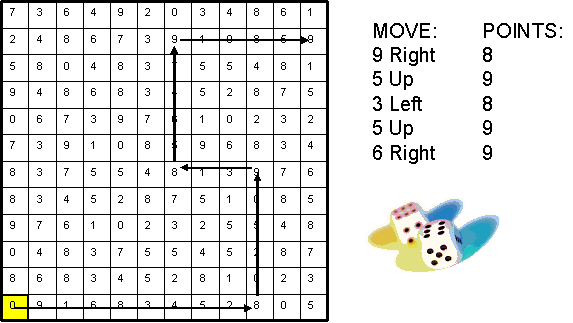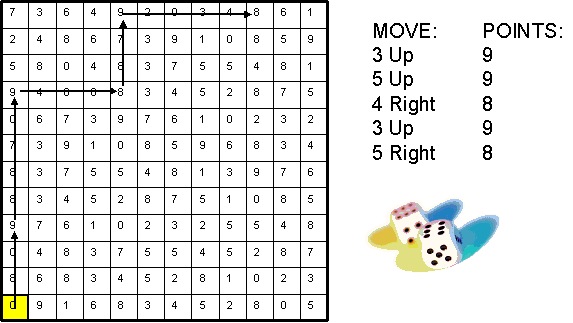## August 25, 2006

...Learn TDD with Codemanship

### Good vs Bad Project Plans

I love dice.

No really, there's a lot you can learn from a pair of dice.

Take yesterday, for example. Yesterday a bunch of people learned the difference between a good project plan and a bad project plan, using a pair of dice (okay, and some pencils and paper).

Here's a game you can play - if you get bored enough... Draw a grid of 12 x 12 squares, and in each square write a random number from 0 - 9. These numbers represent value. Place a counter in the bottom left-hand square. This is where your game will start from.

Take two 6-sided dice. You will throw the dice to move around the grid and earn value from each square you land in.

Before you can play the game, you need to create a plan. You must plan the moves you want to make during the game. Each move is given as a number of squares in one of four directions - right, left, up and down. To successfully complete a move, you must throw the number of squares specified using the sum of the two dice. This means each move must be between 2 and 12 squares.

The aim of the game is to execute the plan and earn as much value as possible using a fixed number of dice throws - in this case, I will allow you 30 throws of the dice.

Consider Plan A, show below:And then consider Plan B:In light of the aim of the game, which is the better plan, and why? What formula could you use to measure the relative effectiveness of such plans? And what the hell has this got to do with project management?

Okay, first thing's first. The better plan is Plan A. Why?

Well, that leads us nicely on to the next question. Think of a sales forecast. A sales team has a pipeline of potential deals. Each deal has an estimated value, and a probability of being completed at a fixed point in time. Let's say we have a deal worth \$100,000, which has a 50% chance of completing in January. So we would forecast sales for January as being \$100,000 * 50% (\$50,000) - or value x probability.

This gives us the possibility of creating a value forecast. A move worth 8 points that requires a me to throw a 7 using two dice (with a probability of 1/6 - 6 ways of throwing a 7 out of 36 possible combinations of 2 dice) would be forecast as 8 * 1/6 = 1.33. A move worth 9 points requiring a 2 (only one way of throwing a 2 using two dice, so that's 1/36) would be forecast as 9 * 1/36 = 0.25.

So, just going on the sum of the forecasts for each plan, Plan A's forecast is significantly higher.

But what about two plans with exactly the same moves with the same values, but in a different order? Is order a factor? Well, yes - order is a big factor. If we are limited to 30 throws, and we put the moves with the highest value forecast at the end of the plan, then what might happen?

More on that in the next posting :-)
Posted 14 years, 11 months ago on August 25, 2006